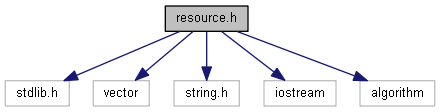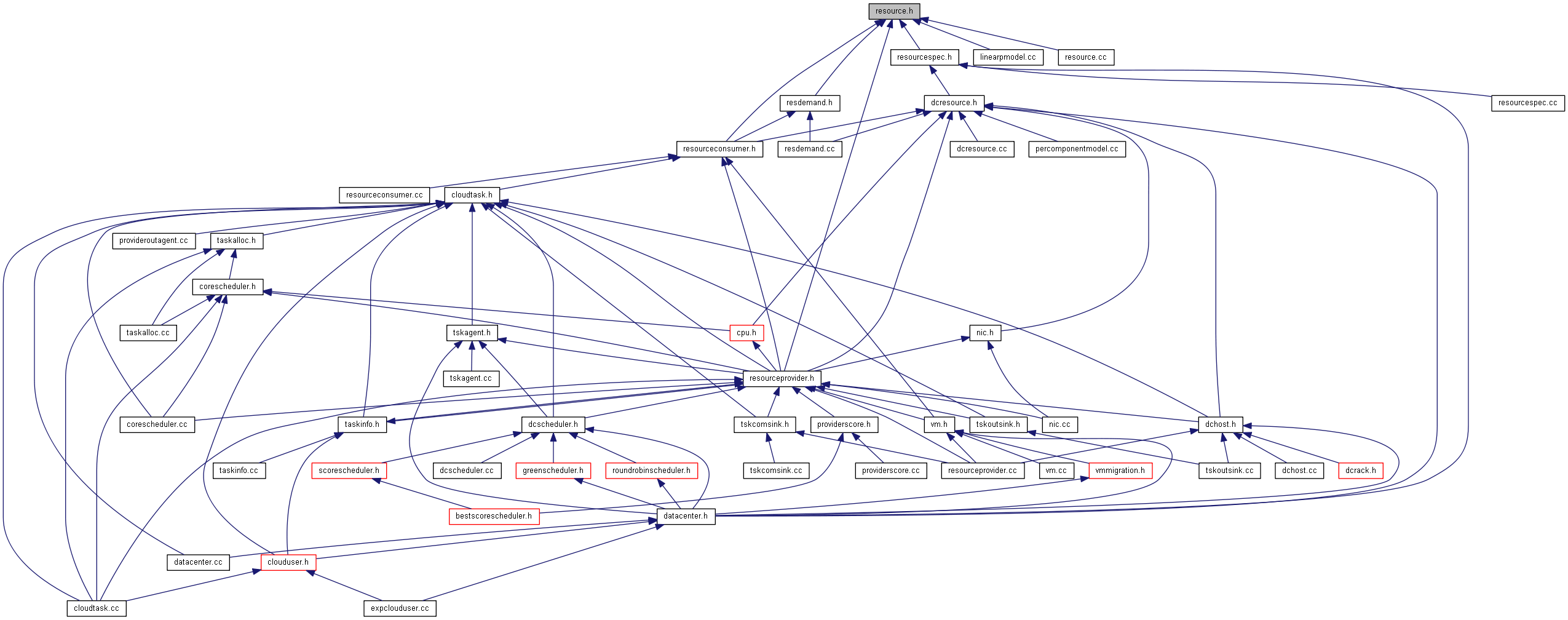GreenCloud Simulator
resource.h File Reference
`#include <stdlib.h>`
`#include <vector>`
`#include <string.h>`
`#include <iostream>`
`#include <algorithm>`
Include dependency graph for resource.h:This graph shows which files directly or indirectly include this file:Go to the source code of this file.

class  Capacity

class  Resource

enum  res_type

## Functions

bool operator== (const Capacity &lhs, const Capacity &rhs)

bool operator!= (const Capacity &lhs, const Capacity &rhs)

bool operator< (const Capacity &lhs, const Capacity &rhs)

bool operator> (const Capacity &lhs, const Capacity &rhs)

bool operator<= (const Capacity &lhs, const Capacity &rhs)

bool operator>= (const Capacity &lhs, const Capacity &rhs)

Capacity operator+ (Capacity lhs, const Capacity &rhs)

Capacity operator- (Capacity lhs, const Capacity &rhs)

bool operator== (const Capacity &lhs, const double &rhs)

bool operator!= (const Capacity &lhs, const double &rhs)

bool operator< (const Capacity &lhs, const double &rhs)

bool operator> (const Capacity &lhs, const double &rhs)

bool operator<= (const Capacity &lhs, const double &rhs)

bool operator>= (const Capacity &lhs, const double &rhs)

Capacity operator+ (Capacity lhs, const double &rhs)

Capacity operator- (Capacity lhs, const double &rhs)

## Enumeration Type Documentation

 enum res_type
Enumerator
Computing
Memory
Storage
Networking
FirstResType
LastResType

Definition at line 19 of file resource.h.

19  {
20  Computing,
21  Memory,
22  Storage,
23  Networking,
25  LastResType = Networking
26 };

## Function Documentation

 bool operator!= ( const Capacity & lhs, const Capacity & rhs )
inline

Definition at line 48 of file resource.h.

48 {return !operator==(lhs,rhs);}
bool operator==(const Capacity &lhs, const Capacity &rhs)
Definition: resource.h:47
 bool operator!= ( const Capacity & lhs, const double & rhs )
inline

Definition at line 65 of file resource.h.

65 {return !operator==(lhs,rhs);}
bool operator==(const Capacity &lhs, const Capacity &rhs)
Definition: resource.h:47
 Capacity operator+ ( Capacity lhs, const Capacity & rhs )
inline

Definition at line 54 of file resource.h.

55 {
56  lhs += rhs;
57  return lhs;
58 }
 Capacity operator+ ( Capacity lhs, const double & rhs )
inline

Definition at line 71 of file resource.h.

72 {
73  lhs += rhs;
74  return lhs;
75 }
 Capacity operator- ( Capacity lhs, const Capacity & rhs )
inline

Definition at line 59 of file resource.h.

60 {
61  lhs -= rhs;
62  return lhs;
63 }
 Capacity operator- ( Capacity lhs, const double & rhs )
inline

Definition at line 76 of file resource.h.

77 {
78  lhs -= rhs;
79  return lhs;
80 }
 bool operator< ( const Capacity & lhs, const Capacity & rhs )
inline

Definition at line 49 of file resource.h.

49 { return (lhs.value < rhs.value);}
double value
Definition: resource.h:33
 bool operator< ( const Capacity & lhs, const double & rhs )
inline

Definition at line 66 of file resource.h.

66 { return (lhs.value < rhs);}
double value
Definition: resource.h:33
 bool operator<= ( const Capacity & lhs, const Capacity & rhs )
inline

Definition at line 51 of file resource.h.

51 {return !operator> (lhs,rhs);}
bool operator>(const Capacity &lhs, const Capacity &rhs)
Definition: resource.h:50
 bool operator<= ( const Capacity & lhs, const double & rhs )
inline

Definition at line 68 of file resource.h.

68 {return !operator> (lhs,rhs);}
bool operator>(const Capacity &lhs, const Capacity &rhs)
Definition: resource.h:50
 bool operator== ( const Capacity & lhs, const Capacity & rhs )
inline

Definition at line 47 of file resource.h.

47 { return (lhs.value ==rhs.value);}
double value
Definition: resource.h:33
 bool operator== ( const Capacity & lhs, const double & rhs )
inline

Definition at line 64 of file resource.h.

64 { return (lhs.value ==rhs);}
double value
Definition: resource.h:33
 bool operator> ( const Capacity & lhs, const Capacity & rhs )
inline

Definition at line 50 of file resource.h.

50 {return operator< (rhs,lhs);}
bool operator<(const Capacity &lhs, const Capacity &rhs)
Definition: resource.h:49
 bool operator> ( const Capacity & lhs, const double & rhs )
inline

Definition at line 67 of file resource.h.

67 {return operator< (rhs,lhs);}
bool operator<(const Capacity &lhs, const Capacity &rhs)
Definition: resource.h:49
 bool operator>= ( const Capacity & lhs, const Capacity & rhs )
inline

Definition at line 52 of file resource.h.

52 {return !operator< (lhs,rhs);}
bool operator<(const Capacity &lhs, const Capacity &rhs)
Definition: resource.h:49
 bool operator>= ( const Capacity & lhs, const double & rhs )
inline

Definition at line 69 of file resource.h.

69 {return !operator< (lhs,rhs);}
bool operator<(const Capacity &lhs, const Capacity &rhs)
Definition: resource.h:49